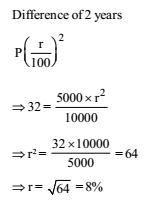# RRB ALP 2018 Practice Test Papers | Arithmetic Questions (Day-30)

Dear Aspirants, Here we have given the Important RRB ALP & Technicians Exam 2018 Practice Test Papers. Candidates those who are preparing for RRB ALP 2018 can practice these Arithmetic Questions to get more confidence to Crack RRB 2018 Examination.

[WpProQuiz 1994]

Click “Start Quiz” to attend these Questions and view Explanation

1. The sum of two numbers is 24 and their product is 143. The sum of their squares is

(a) 296

(b) 295

(c) 290

(d) 228

1. A boy found that the average of 20 numbers is 35 when he writes a number ’61’ instead of ’16’. The correct average of 20 numbers is

(a) 32.75

(b) 37.25

(c) 34.75

(d) 34.25

1. The average of some natural numbers is 15. If 30 is added to first number and 5 is subtracted from the last number the average becomes 17.5 then the number of natural number is:

(a) 20

(b) 30

(c) 15

(d) 10

1. The salary of an employee increases every year in the month of July by 10%. If his salary in May 2000 was Rs. 15,000, his salary in October 2001 was

(a) Rs. 16,500

(b) Rs. 18,000

(c) Rs. 18,150

(d) Rs. 19,965

1. In a motor of 120 machine parts, 5% parts were defective. In another motor of 80 machine parts, 10% parts were defective. For the two motors considered together, the percentage of defective machine parts were

(a) 7

(b) 6.5

(c) 7.5

(d) 8

1. The difference between the compound interest and simple interest for the amount Rs. 5,000 in 2 years is Rs. 32. The rate of interest is

(a) 5%

(b) 8%

(c) 10%

(d) 12%

1. A man leaves Rs. 12,600 to be divided among 7 sons, 3 daughters and 5 nephews. If each daughter receives three times as much as each nephew and each son seven times as much as each nephew, then each daughter’s share is

(a) Rs. 700

(b) Rs. 650

(c) Rs. 600

(d) Rs. 750

1. A man walking at the rate of 5 km/h crosses a bridge in 15 minutes. The length of the bridge (in metres) is:

(a) 600

(b) 750

(c) 1000

(d) 1250

1. Two-third of one-seventh of a number is 87.5% of 240. What is the number?

(a) 2670

(b) 2450

(c) 2205

(d) 1470

1. 10. What is the remainder when 496 is divided by 6?

(a) 0

(b) 2

(c) 3

(d) 4

Let the two numbers be x and y.

x + y = 24 and, xy = 143

x2 + y2 = (x + y)2 – 2xy = (24)2 – 2 × 143 = 576 – 286 = 290

Sum of 20 numbers = 20 × 35 = 700

Sum of 20 numbers when 61 is replace by 16 => 700 – 61 + 16 = 655

Correct average of 20 numbers = 655/20= 32.75

Let number of natural numbers be x

15x + 30 – 5 = 17.5 x

25 = 2.5x

x = 25/2.5=10

So 10 natural numbers are there.

Salary in May 2000 = Rs. 15000

Salary in July 2000 => 15000 + 10% of 15000 = Rs.16500

Salary in October 2001 = 16500 + 10% of 16500 = Rs.18150

Total defective part = 5 /100 ×120 +10/100 × 80 = 6 + 8 = 14

Defective% = 14 ×100 = 7%

1. Answer: (b)7S + 3D + 5N = Rs. 12600… (1)

According to Question

D = 3N

S = 7N

Putting these values in equation (1)

49 N + 9N + 5N = 12600

=> 63N = 12600

=> N = 200

Each daughter’s share = 3 × Nephew’s share = 3 × 200 = Rs. 600

Speed = 5×5/18= 25/18 5 m/ sec

Distance covered in 15 minutes = 25/18x 15x 60 m= 1250 m.

Let number be x then,

2/3×1/7*x=240×87.5/100= x = 2205

Let us divide the different powers of 4 by 6 and find the remainder.

So remainder for 41 = 4, 42 = 4, 43 = 4, 44 = 4, 45 = 4, 46 = 4 and so on.

Hence remainder for any power of 4 will be 4 only.

RRB ALP 2018 Practice Test Papers | Arithmetic Questions (Day-1)

RRB ALP 2018 Practice Test Papers | Arithmetic Questions (Day-2)

RRB ALP 2018 Practice Test Papers | Arithmetic Questions (Day-3)

RRB ALP 2018 Practice Test Papers | Arithmetic Questions (Day-4)

RRB ALP 2018 Practice Test Papers | Arithmetic Questions (Day-5)

RRB ALP 2018 Practice Test Papers | Arithmetic Questions (Day-6)

RRB ALP 2018 Practice Test Papers | Arithmetic Questions (Day-7)

RRB ALP 2018 Practice Test Papers | Arithmetic Questions (Day-8)

RRB ALP 2018 Practice Test Papers | Arithmetic Questions (Day-9)

RRB ALP 2018 Practice Test Papers | Arithmetic Questions (Day-10)

RRB ALP 2018 Practice Test Papers | Arithmetic Questions (Day-11)

RRB ALP 2018 Practice Test Papers | Arithmetic Questions (Day-12)

RRB ALP 2018 Practice Test Papers | Arithmetic Questions (Day-13)

RRB ALP 2018 Practice Test Papers | Arithmetic Questions (Day-14)

RRB ALP 2018 Practice Test Papers | Arithmetic Questions (Day-15)

RRB ALP 2018 Practice Test Papers | Arithmetic Questions (Day-16)

RRB ALP 2018 Practice Test Papers | Arithmetic Questions (Day-17)

RRB ALP 2018 Practice Test Papers | Arithmetic Questions (Day-18)

RRB ALP 2018 Practice Test Papers | Arithmetic Questions (Day-19)

RRB ALP 2018 Practice Test Papers | Arithmetic Questions (Day-20)

RRB ALP 2018 Practice Test Papers | Arithmetic Questions (Day-21)

RRB ALP 2018 Practice Test Papers | Arithmetic Questions (Day-22)

RRB ALP 2018 Practice Test Papers | Arithmetic Questions (Day-23)

RRB ALP 2018 Practice Test Papers | Arithmetic Questions (Day-24)

RRB ALP 2018 Practice Test Papers | Arithmetic Questions (Day-25)

RRB ALP 2018 Practice Test Papers | Arithmetic Questions (Day-26)

RRB ALP 2018 Practice Test Papers | Arithmetic Questions (Day-27)

RRB ALP 2018 Practice Test Papers | Arithmetic Questions (Day-28)

RRB ALP 2018 Practice Test Papers | Arithmetic Questions (Day-29)